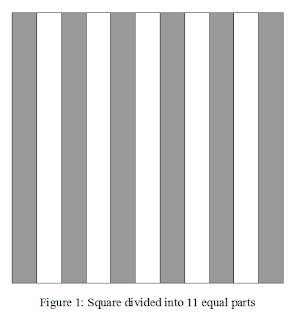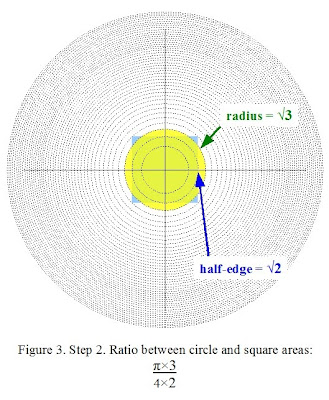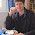## Sunday, March 21, 2010

### Exhaustion of nested squares and Wallis productWhen one is asked to divide a square into N equal area parts, we commonly think of dividing a square into N equal rectangles. Figure 1 shows an example for N=11.

There is another general solution to that problem, in particular we could wrap it up in an enigma: is it possible to divide a square into N square-shaped parts of equal area, N being any integer?

The nested circles structure described in Figure 4 of the Variations of circular area division into equal parts post suggests the following solution.

Step 1. Starting from a circle of radius 1, area π, draw circles of incrementing area 2π, 3π, 4π, 5π, 6π, and so on... The resulting concentric rings have all the same area π.Step 2. For each circle, circumscribe a square. The resulting squares have area 4, 8, 12, 16, 20, 24, and so on...Step 3. Conclusion: the areas between each nested squares are equal. The figure illustrates the case for N=11.So here we have a compass and ruler solution for dividing a square into N equal area parts. An interesting corollary is that this division into N equal parts can be applied to any 2D-figure, whether it be a triangle, a pentagon, any polygon, or even arbitrary drawings, provided that it be circumscribed (or inscribed) in a circle. As an answer to a comment on the preceding post, why not divide Leonardo da Vinci's Vitruvian man into N nested Vitruvian men? There are certainly other applications, one of them has been suggested to me by another comment on the preceding post.

In order to calculate an approximate value of π, Archimedes used a method of exhaustion which consists in inscribing a circle between two identically shaped polygons. Increasing gradually the number of sides of the polygon, the shape of the polygon approaches the shape of a circle. This geometrical approach is at the origin of his numerical approximation of π. Many other ways have been developed to approximate π, especially using infinite series. Geometrical representations of these series are rarely available, probably because no direct link has been found between them and the circle. The benefit of the nested circle and square structure of Figure 2 is that it suggests alternative methods of exhaustion.

For example, we could try to equate the area of a circle to the area of a square in this nested structure. This yields an approximation for squaring the circle (or circling the square). For a circle of radius 1, the area of the circle is π, the area of the circumscribed square is 4. The ratio between both areas is therefore π/4. The procedure to let that ratio go to 1 then follows the following steps.

Step 1. Draw the initial circle and square with ratio π/4.
Step 2. A better fit would be to inscribe both figures in higher orders of the nested structure. But the area of the circle is too small with respect to the square. So, we must enlarge the area of the circle a little more than the square. We therefore enlarge the area of the circle by 3 while enlarging the area of the square by 2, which is illustrated in the figure below.Step 3. But now the area of the circle is too large with respect to the square. So for this fit, we must enlarge the area of the circle a little less than the area of the square. We therefore enlarge the area of the circle by 3, while enlarging the area of the square by 4, as illustrated below.Step 4. But now the area of the circle is again too small with respect to the square. So for the next fit, we must enlarge the area of the circle a little more than the area of the square. We therefore enlarge the area of the circle by 5, while enlarging the area of the square by 4, as illustrated below.
Step 5. As after step 2, the area of the circle is too large with respect to the square... and I become a bit exhausted by drawing at exponentially growing scales;-) but I hope I've shown enough to suggest the continuation of this exhaustion scheme. You can of course rescale the figures, keeping track of the successive numbers and their roots on the figures.

The described method of exhaustion searches for a limit by alternately letting the circular area become larger, smaller, larger, smaller, etc... than the square area, but each time with a reduced fraction. As a limit it can be expressed by the following equation:It seems we have gone through Wallis' product, which is usually disconnected from any geometrical approach.
______________________________________________________

Profound study of nature is the most fertile source of mathematical discoveries. ~ Joseph Fourier

1.Hi Arjen,

I can see the wheels are turning or should I say the circles :-) Levity aside some of best ideas regarding mathematics, which do or may relate to our physical world, came as a result of such thinking, as to imagine geometrically how things come to be as they are. The circle of course probably being the best example of them all, combined with its three dimensional offspring being the sphere.

What I find interesting in your first demonstrated analysis, that being besides what you mentioned, is what you end up with is a perspective rendering of a pyramid viewed from above. So it has us to wonder as we iterate in if we are also not interating up or perhaps down, as to have another dimension or rather partial (fractal) dimension come to be realized.

As it happens others, first beginning with those such as Von Koch, with his snowflake would have the concept of fractal dimensioning, as you give light to here, have us come to conclusions about dimensions not being of increments of only whole numbers yet also as fractions of the whole. Of course Benoit Mandelbrot would later discover this could be extended to the the complex number plane , where mathematical concepts like imaginary numbers come to be better understood as not rightfully referred to being imaginary. This has then had it easier for me to imagine where all those digits of PI could possible reside, if they only being restricted to existing in one dimension as it is the norm to have it so conceptualized.

So then I’ve often wondered if Archmedes could ever imagined how his first thought born of drawing circles in the sand would lead in terms of our ever broadening undertstanding of mathematics yet the world itself more generally.

Best,

Phil

2.Hi Phil,

That downward perspective rendering of a pyramid is indeed interesting. Friends around me also pointed me towards that, when I showed them that figure. Concerning fractals, it seems indeed that nature is full of recurrent patterns from which we gain insight. I'll have to look closer at works from Von Koch ans Mandelbrot.

Besides, as a complement to my post, I realized today that there is another geometrical representation for Wallis product. In fact I read it a week ago at a very entertaining blog, but I didn't realize at that time that it was Wallis' product, because I was only beginning to learn about it.

3.Hi,
1.